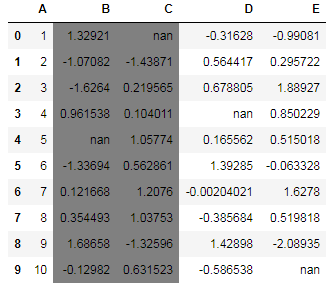﻿ Pandas styling: Write a Pandas program to highlight dataframe's specific columns - w3resource# Pandas styling Exercises: Write a Pandas program to highlight dataframe's specific columns

## Pandas styling: Exercise-7 with Solution

Create a dataframe of ten rows, four columns with random values. Write a Pandas program to highlight dataframe's specific columns.

Sample Solution :

Python Code :

``````import pandas as pd
import numpy as np
np.random.seed(24)
df = pd.DataFrame({'A': np.linspace(1, 10, 10)})
df = pd.concat([df, pd.DataFrame(np.random.randn(10, 4), columns=list('BCDE'))],
axis=1)
df.iloc[0, 2] = np.nan
df.iloc[3, 3] = np.nan
df.iloc[4, 1] = np.nan
df.iloc[9, 4] = np.nan
print("Original array:")
print(df)
def highlight_cols(s):
color = 'grey'
return 'background-color: %s' % color
print("\nHighlight specific columns:")
df.style.applymap(highlight_cols, subset=pd.IndexSlice[:, ['B', 'C']])
``````

Original array:

```Original array:
A         B         C         D         E
0   1.0  1.329212       NaN -0.316280 -0.990810
1   2.0 -1.070816 -1.438713  0.564417  0.295722
2   3.0 -1.626404  0.219565  0.678805  1.889273
3   4.0  0.961538  0.104011       NaN  0.850229
4   5.0       NaN  1.057737  0.165562  0.515018
5   6.0 -1.336936  0.562861  1.392855 -0.063328
6   7.0  0.121668  1.207603 -0.002040  1.627796
7   8.0  0.354493  1.037528 -0.385684  0.519818
8   9.0  1.686583 -1.325963  1.428984 -2.089354
9  10.0 -0.129820  0.631523 -0.586538       NaN

Highlight specific columns:
```

Sample Output:

``````

Python Code Editor:

Have another way to solve this solution? Contribute your code (and comments) through Disqus.

What is the difficulty level of this exercise?

﻿

## Python: Tips of the Day

Python: Annotated Assignment Statement

This might not seem as impressive as some other tricks but it's a new syntax that was introduced to Python in recent years and just good to be aware of.

Annotated assignments allow the coder to leave type hints in the code. These don't have any enforcing power at least not yet. It's still nice to be able to imply some type hints and definitely offers more options than only being able to comment regarding expected types of variables.

```day: str = 'Monday'
print(day)
lst: list = [1,2,3,4]
print(lst)
```

Output:

```Monday
[1, 2, 3, 4]```

Or the same thing in a shorter way:

```day= 'Monday' #str
print(day)
lst= [1,2,3,4] # list
print(lst)
```

Output:

```Monday
[1, 2, 3, 4]```